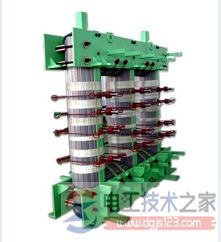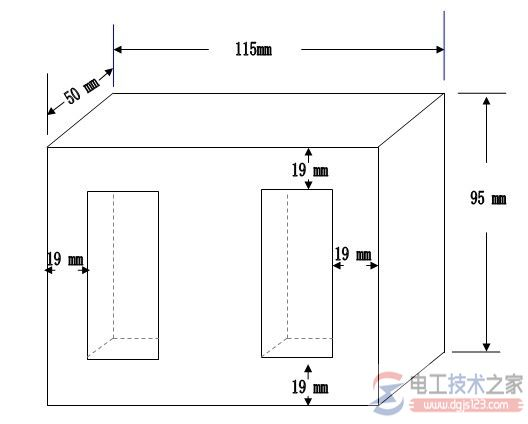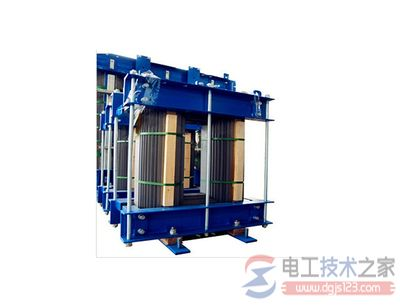`>`首页 > 电工基础 > 变压器`>`变压器铁芯截面积的计算公式 正文

# 变压器铁芯截面积的计算公式及问题解答

###### 来源：电工天下 编辑：小电工发布时间：2016-05-15 16:05:461KVA以内的变压器一般采用EI型铁芯的壳式结构，其铁芯截面积按下式计算。

K为经验系数，其值为

S=ab(cm2)

a为铁芯宽(cm)
b为铁芯净迭厚(cm)

b'=b/ks(cm)1、铁芯截面积S=9X7.2=65cm2)
2、根据铁芯截面积(cm2)求容量
P=(S/1.25)2（VA）=(65/1.25)2=2700VA
3 根据容量确定一次线圈和二次线圈的电流
I=P/U
I单位A、P单位vA、U单位v.
I1=P/U=2700/220=12A
I2=P/U=2700/40=67.5A（电压取40V，是以工作时的电压算）
4、每伏匝数
ωo=45/S=45/65=0.7匝
5、一、二次匝数
初级 ω1=U1ωo=220X0.7=154匝
次级 ω2=1.05 U2ωo =1.05X60X0.7≈44匝（电压取60V，是以空载时的电压算）
6、求导线直径（截面积）
初级  d1=0.72√I (根号I1）=0.72√12≈ 2.5mm（4mm2或6mm2铜线都可以）
次级  d2=0.72√I (根号I2）=0.72√67.5≈ 6mm（16mm2或25mm2铜线都可以）
经常长时间用取大值。

ee型骨架 加个图更好  铁芯外长5.6cm 宽 4.8cm  迭厚2.5cm ，内长  2.8宽  1cm  (有两个) 怎么算出它的有效面积？

ee型骨架铁芯外长 L1 =5.6cm ，宽 W1 =4.8cm  迭厚D =2.5cm ；内长 L2 = 2.8cm，宽W2=  1cm  (有两个) 它的有效面积S 计算如下：

S = （L1 - L2）x D = (5.6-2.8)x2.5 = 7 cm2

`变压器铁芯截面积的计算公式相关的文章`